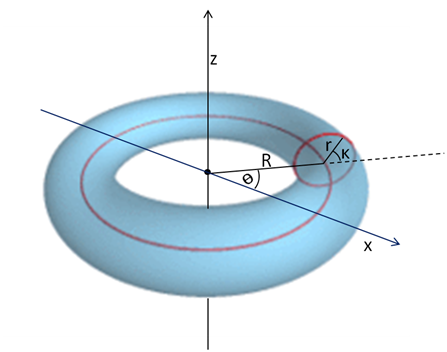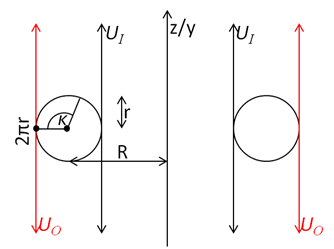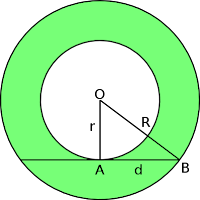## Question

Show that the two-dimensional torus T2 is a manifold, by explicitly constructing an appropriate atlas. (Not a maximal one, obviously).Fig 1: A Torus, with help from Wikipedia

We can define a torus as points in R3 that lie on the surface created by rotating a small circle of radius r round a larger circle of radius R. The larger circle is in the X-Y plane. Any point on the torus can be given by (θ , κ) as shown in Fig 1. There are no limits on θ , κ.

I realised that I could map to infinite cylinders touching the inner and outer edges of the torus and from them to annuli as in Exercise 2.01. No doubt our cunning author had set this trap. Having been on that wild goose chase, I came to a linear map from T2 to the annuli and realised that we can also map from T2 to two open rectangles much as one can map from S1 to R1. Fig 2 shows a section at some θ through our torus. We almost have the two charts UO (Outer) and UI (Inner) we just need to cut them and unroll them to make open rectangles. We cut the outer UO at θ = 0 and the inner UI at θ = -π. If we cut them both at the same θ it would lead to disaster.Fig 2: Section through torus and rectangular maps.
This is where it gets complicated and we end up with a few maps, so;Fig 4: Showing the transformations. T2 is exactly UI  ∩ UO.
We now want to check that the charts obey the strict conditions, as given in the book before Fig 2.13 at the bottom of page 29, to make an atlas from T2 to R2. I have adapted them for the exercise.

1. "The union of the charts UI  , UO is equal to T2; that is, UI  , UO cover T2."
We have two charts UI  , UO; their union manifestly covers T2 by construction.
We could check that there are no cracks in the union by combining the limits on ϕI  , ϕO. We have  -π< θ<2π and -π<κ<2π. That covers T2.

2. "The charts are smoothly sewn together. More precisely, if two charts overlap, UIUO ≠∅, then the map (ϕI ∘ ϕO-1) takes points in ϕO (UIUO  ) ⊂  R2 onto* an open set ϕI (UIUO ) ⊂ R2, and all these maps must be C. The reverse for  (ϕ∘ ϕI-1) also applies.

* We remind ourselves that onto means each point of the target has at least one point of the source mapped onto it.

The first part was easy as shown. The second part was fiddly and you can read the detail and wild goose chase in Ex 2.03 2D Torus is a manifold.pdf. It has helped me greatly to understand manifolds.

## Sunday, 19 August 2018

Sadly on 19th August I accidentally deleted all the files in my Space time and geometry. I then recovered them from the Microsoft bin. That was a mistake because it changed the URLs of all the files in the directory, so many links prior to this date are broken. If you need to find a file whose link is broken please look at the List of All File link in the LINKS section. Read more for how how to avoid this in future.

## Question

Just because a manifold is topologically non-trivial doesn't necessarily mean it can't be covered by a single chart. In contrast to the circle S1, show that the infinite cylinder R × S1 can be covered by just one chart, by explicitly constructing the map.

The first thing about the chart is that it must be open. That is its boundary is not included. The reason this is important is because the chart must be differentiable everywhere and if any boundary is included, it will not be differentiable there. It would be a sharp edge. This is why S1 cannot be mapped on one chart.Fig 1

Any point on the infinite cylinder can be given coordinates t, θ. t is the distance above or below some arbitrary point, with values from −∞ to +∞ . θ is the angle round the cylinder. 0 ≤ θ < 2π. We cannot simply unroll the cylinder because then we would get an infinitely long strip closed on the left an open on the right as shown in figure 1.

So I started by trying to map to a line which spiralled up the cylinder at some very small angle. Any point on the cylinder would map onto the line and vice-versa. This didn't seem to work.

### Physics forums solution

The first was on physics forums from July 2016 atAnnulus. Wikipedia

Map each line on the cylinder that runs parallel to the cylinder's axis of rotation, to a radial line in the annulus. Then set units along that radial line by parameterizing it in such a way that one approaches but never reaches the outer (inner) circumference of the annulus as t  (t−∞). The parameter t can represent signed distance along the line on the cylinder from an arbitrarily chosen starting point.

This solution might seem to suffer from the problem with the circle S1: Two charts are needed to map it. However this may not the case as I showed in "Commentary 2.2 Covering by a single chart".

Following andrewkirk's instructions we could use the tangent function to parameterise the line as shown belowFig 3. Section through infinite cylinder, showing tangent curve (blue),  and mappings of three points on the cylinder (green)
The tangent curve will rotate through 2π to form a very tall wine glass, without a base and a hole at the bottom. Our original cylinder has radius r and an outer cylinder has a radius R. It limits the outward extent of the curve. The equation of the curve is
t=tan((x- (R+r)/2) π / (R-r))    (1)
It is not trivial to derive the equation. Click Read more below, if you care.

Consider the point P at (t , θ) which maps to P' at (x , θ). From (1)
x = (R+r)/2 +(R-r) (tan-1t) / π   (2)

Therefore, more conventionally, the mapping is
θ' = θ
t' = (R+r)/2 +(R-r) (tan-1t) / π
It is easy to show that this is C. (see pdf for details.)

The most interesting thing about this is that it shows that one can map an infinite area (the cylinder) onto a finite open area - the annulus. It is quite easy to imagine deforming the cylinder into the annulus. The 'mapping' from infinity to the open boundary is also interesting and adds to our intuitive understanding of why an open boundary is differentiable and a closed boundary (or sharp edge) is not.

### University of California Santa Barbara solution

The second solution was at University of California Santa Barbara saved here.
It also contains and answer to Exercise 1.04 on super luminal velocity and I discovered a small treasure trove of answers which I have catalogued in Answers to Exercises..

The answer for the infinite cylinder starts with a half cylinder and ends with a punctured plane. It is more complicated. It should be noted that a punctured plane has striking similarities to an open annulus.

For my pdf on this subject with prettier equations and further links look here
Ex 2.01 One chart for infinite cylinder.pdf

## Wednesday, 15 August 2018

### Commentary 2.2 Covering by a single chart

I was thinking about "Exercise 2.01 One chart for infinite cylinder". I could not understand why the Physics Forums solution by andrewkirk which invokes an annulus did not suffer from the same problem as a circle S1. How could you map an infinite annulus to one chart, when the angle round the annulus must be measured and is 0 ≤ θ < 2π. So the coordinate θ cannot be mapped onto an open chart in R1.

This is a similar question to asking why the plane in polar coordinates can be mapped on to one R2 chart. Or the reverse that the plane in polar coordinates can not be mapped on to one R2 chart. That last sentence is obviously not true.R2 in polar coordinates
The polar coordinates are r ≥ 0
and  θ where  0 ≤ θ < 2π
Neither of the coordinates are open on R1.

The mapping of polar coordinates to R2 is well known:
x = r cos θ, y = r sin θ.
So there is a mapping from the plane in polar coordinates to R2.

#### Conclusion

Coordinates on a manifold may not map onto charts in R1 but this does not stop the manifold mapping onto a chart.

Therefore it may be safe to use the infinite open annulus to construct our chart for the infinite cylinder.

Available as pdf

## Question

Verify the claims made about the commutator of two vector fields at the end of section 2.3 (linearity, Leibnitz, component formula, transformation as a vector field).
The commutator of two vector fields X and Y is defined by its action on a function f(xμ) as
 [X,Y](f) ≡ X(Y(f))) - Y(X(f))) (2.20)

1) Linearity: if f and g are functions and a, b real numbers, the commutator is linear:
 [X,Y](af + bg) = a[X,Y](f) + b[X,Y](g) (2.21)
2) It obeys the Leibnitz rule:
 [X,Y](fg) = f[X,Y](g) + g[X,Y](f) (2.22)
3) The components of the vector field [X,Y]μ are given by:
 [X,Y]μ = Xλ∂λYμ - Yλ∂λXμ (2.23)
4) Transformation as a vector field:
Perform explicitly a coordinate transformation on the expression (2.23) to verify that all potentially non-tensorial pieces cancel and the result transforms like a vector field.

The first two were pretty easy. They just involved using the definition, using the fact that X and Y must be linear and / or obey the Leibnitz rule and then cancelling and / or using the definition again. My answers are almost line for line in agreement with the UCSB solution.

The third was a bit more difficult. I used the fact that the vector field is also a derivative operator. There were two slightly dubious steps in my proof: thaY(xλ )=Yλ and removing  entirely to get equation 0.5. But I think they are OK especially as they give the right answer!

I eventually understood the fourth question and knew where to start and where it should end. The four fairly hairy steps in between eluded me. I have followed the steps in detail in my pdf.

The UCSB solutions also proved the Jacobi identity for vector fields. It is

[ [X, Y ], Z ] + [ [Y, Z], X ] + [ [Z, X], Y ] = 0.
The proof was easy!

Ex 2.04 Commutator of two vector fields.pdf

## Tuesday, 7 August 2018

### Commentary 2.3 Coordinate transformations plane and sphere

The last part of Exercise 2.04 asks us to perform a coordinate transformation. We really want a transformation from a curved space to a curved space, so I decided to try to find a coordinate transformation for the surface of a sphere. This was quite hard. So I started with rotating the plane, R2. That came in useful later to calculate the transformation for a sphere.
Consider a point C which has coordinates (x , y) in S and (x' , y') in S', S' is rotated by an angle θ to S. It's quite easy to work out x' and y' from x and y and the answer was given at (1.3.1) in the book.

I then wanted to get a coordinate transformation for the surface of a sphere. The coordinates are longitude, latitude as usual and the rotated coordinates have 0 latitude rotated up by θ along 0 longitude as pictured below.
First of all I tried to do this directly like the rotating plane. But I got in a mess. I then decided to combine three transformations:
A: Transform polar coordinates into Cartesian: S -> S"
B: Rotate the Cartesian coordinates: S" -> S"' (already done for plane)
C: Convert back to polar coordinates: S"' -> S'